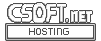Agar 1.7 Manual

(Printable Version)
M_Geometry(3)

# SYNOPSIS

```#include <agar/core.h>
#include <agar/gui.h>
#include <agar/math/m.h>
```

# DESCRIPTION

The Agar-Math library implements a number of algorithms and operations on related to geometrical structures. The following basic structures are defined, see their respective manual pages for more details:
 M_Line(3) Line segment or ray in R2 (M_Line2) or R3 (M_Line3). M_Circle(3) Circle in R2 (M_Circle2) or R3 (M_Circle3). M_Sphere(3) Sphere in R3. M_Plane(3) Plane in R3 (M_Plane). M_Triangle(3) Triangle in R2 (M_Triangle2) or R3 (M_Triangle3). M_Rectangle(3) Rectangle in R2 (M_Rectangle2) or R3 (M_Rectangle3). M_Polygon(3) Polygon in R2. M_Polyhedron(3) Polyhedron in R3.

# GENERIC GEOMETRIC ENTITIES ↑

A number of routines (e.g., computation of intersections) return sets of zero or more geometrical structures of different types, depending on the

The M_Geom2 structure defines a geometrical entity in R2:
```typedef struct m_geom2 {
M_GeomType type;
union {
M_Vector2    point;
M_Line2      line;
M_Circle2    circle;
M_Triangle2  triangle;
M_Rectangle2 rectangle;
M_Polygon    polygon;
} g;
} M_Geom2;
```

Similarly, M_Geom3 defines an entity in R3:
```typedef struct m_geom3 {
M_GeomType type;
union {
M_Vector3    point;
M_Line3      line;
M_Circle3    circle;
M_Triangle3  triangle;
M_Rectangle3 rectangle;
M_Polygon    polygon;
M_Sphere     sphere;
M_Plane      plane;
M_Polyhedron polyhedron;
} g;
} M_Geom3;
```

The type field can take on the following values:
 M_NONE Undefined type M_POINT An M_Vector2(3) or M_Vector3(3). M_LINE An M_Line2(3) or M_Line3(3). M_CIRCLE An M_Circle2(3) or M_Circle3(3). M_POLYGON An M_Polygon(3). M_TRIANGLE An M_Triangle2(3) or M_Triangle3(3). M_RECTANGLE An M_Rectangle2(3) or M_Rectnagle3(3). M_SPHERE An M_Sphere(3). M_PLANE An M_Plane3(3).

# GENERIC GEOMETRIC ENTITIES: SETS ↑

Sets of generic geometric entities in R2 are described by the structure:
```typedef struct m_geom_set2 {
M_Geom2 *g;
Uint n;
} M_GeomSet2;
```
Similarly for R3:
```typedef struct m_geom_set3 {
M_Geom3 *g;
Uint n;
} M_GeomSet3;
```

The following routines allow the manipulation of these sets.

M_GeomSet2 M_GEOM_SET_EMPTY (void)

M_GeomSet3 M_GEOM_SET_EMPTY (void)

void M_GeomSetAdd2 (M_GeomSet2 *S, const M_Geom2 *g)

void M_GeomSetAdd3 (M_GeomSet3 *S, const M_Geom3 *g)

void M_GeomSetFree2 (M_GeomSet2 *S)

void M_GeomSetFree3 (M_GeomSet3 *S)

The M_GEOM_SET_EMPTY() macro is provided as a static initializer for M_GeomSet2 and M_GeomSet3.

The M_GeomSetAdd2() and M_GeomSetAdd3() functions insert a copy of the entity g into the geometric set S.

M_GeomSetFree2() and M_GeomSetFree3() release the resources allocated by a geometric set.